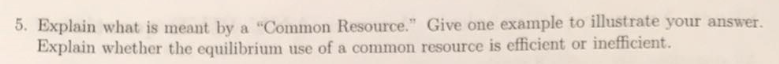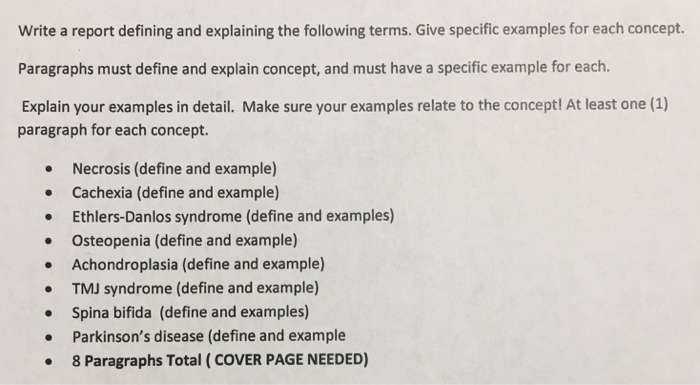Question

# Define the various state of a resource in simulation modelling and give an example of each...

Define the various state of a resource in simulation modelling and give an example of each (Explain in detail)

We need at least 10 more requests to produce the answer.

0 / 10 have requested this problem solution

The more requests, the faster the answer.

All students who have requested the answer will be notified once they are available.

#### Earn Coins

Coins can be redeemed for fabulous gifts.

Similar Homework Help Questions
• ### 1)Define and give an example of a common resource. Without government intervention, will people use this...

1)Define and give an example of a common resource. Without government intervention, will people use this good too much or too little? Why? 2)  Define and give an example of a public good. Is it likely that the private market provide this good on its own? Explain.

• ### Explain in detail. In Simulation Modelling. What is goodness of fit test and when do you...

Explain in detail. In Simulation Modelling. What is goodness of fit test and when do you use such a tool?

• ### Distinguish between data analysis, hypothesis testing modelling and estimation. Give a simple example of each and...

Distinguish between data analysis, hypothesis testing modelling and estimation. Give a simple example of each and discuss the appropriate contexts in which each should be used.

• ### Define detail reports, exception reports, and summary reports and give an example of each for a...

Define detail reports, exception reports, and summary reports and give an example of each for a payroll system.

• ### What is the difference between simulation and optimization? Give practical example for each in field of...

What is the difference between simulation and optimization? Give practical example for each in field of civil engineering or construction management.

• ### 5. Explain what is meant by a "Common Resource." Give one example to illustrate your answer....5. Explain what is meant by a "Common Resource." Give one example to illustrate your answer. Explain whether the equilibrium use of a common resource is eficient or inefficient.

• ### Define exteroceptors and interoceptors. Give a possible example of each. Explain the international standard used for...

Define exteroceptors and interoceptors. Give a possible example of each. Explain the international standard used for evaluating hearing impairment and how it is used for calculations. What is a simple way conduction deafness can be simulated? Explain how this simulates conduction deafness. Is there a simple way to simulate nerve deafness? Why or why not?

• ### The following are GASB’s fund balance classifications. Define and give an example of each: Non-spendable, Restricted,...

The following are GASB’s fund balance classifications. Define and give an example of each: Non-spendable, Restricted, Committed, Assigned, and Unassigned. Define and give an example of each of GASB's fund balance classifications- Non Spendable, Restricted, Committed, Assigned, and Unassigned

• ### Write a report defining and explaining the following terms. Give specific examples for each concept. Paragraphs...Write a report defining and explaining the following terms. Give specific examples for each concept. Paragraphs must define and explain concept, and must have a specific example for each. Explain your examples in detail. Make sure your examples relate to the concept! At least one (1) paragraph for each concept. Necrosis (define and example) Cachexia (define and example) Ethlers-Danlos syndrome (define and examples) Osteopenia (define and example) Achondroplasia (define and example) TMJ syndrome (define and example) Spina bifida (define and...

• ### Explain association, aggregation and composition relationships of class-based modelling approach with one-one suitable example [no need...

Explain association, aggregation and composition relationships of class-based modelling approach with one-one suitable example [no need to write any code]. Represent each relationship via UML diagram? What will be the impact of low cohesion in class-based modelling?